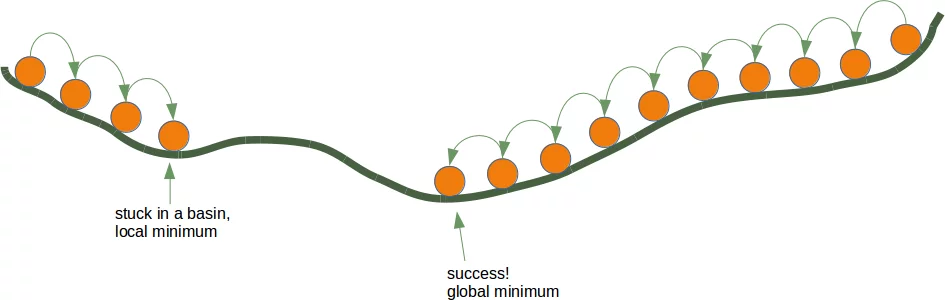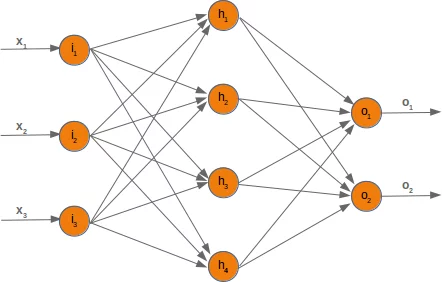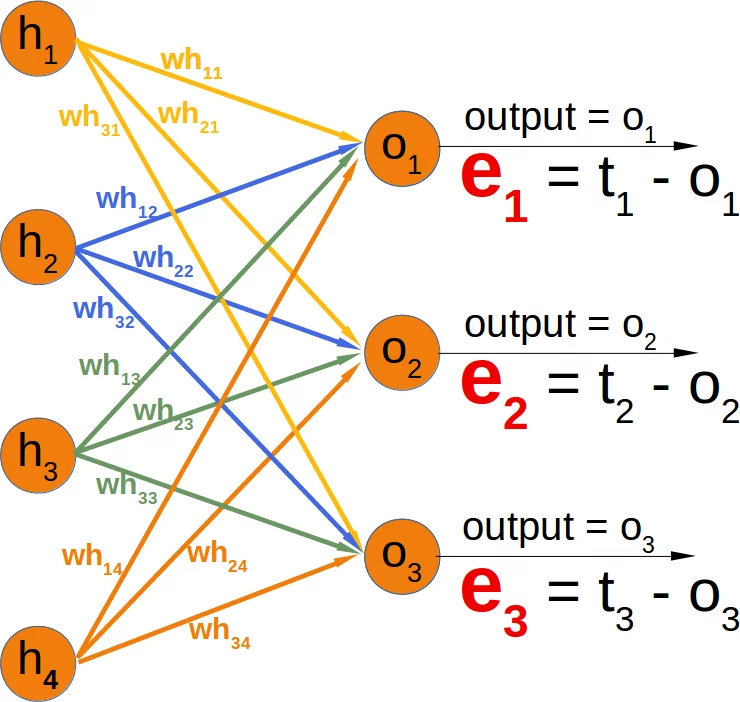## Backpropagation in Neural Networks

### IntroductionWe already wrote in the previous chapters of our tutorial on Neural Networks in Python. The networks from our chapter Running Neural Networks lack the capabilty of learning. They can only be run with randomly set weight values. So we cannot solve any classification problems with them. However, the networks in Chapter Simple Neural Networks were capable of learning, but we only used linear networks for linearly separable classes.

Of course, we want to write general ANNs, which are capable of learning. To do so, we will have to understand backpropagation. Backpropagation is a commonly used method for training artificial neural networks, especially deep neural networks. Backpropagation is needed to calculate the gradient, which we need to adapt the weights of the weight matrices. The weight of the neuron (nodes) of our network are adjusted by calculating the gradient of the loss function. For this purpose a gradient descent optimization algorithm is used. It is also called backward propagation of errors.

Quite often people are frightened away by the mathematics used in it. We try to explain it in simple terms.

Explaining gradient descent starts in many articles or tutorials with mountains. Imagine you are put on a mountain, not necessarily the top, by a helicopter at night or heavy fog. Let's further imagine that this mountain is on an island and you want to reach sea level. You have to go down, but you hardly see anything, maybe just a few metres. Your task is to find your way down, but you cannot see the path. You can use the method of gradient descent. This means that you are examining the steepness at your current position. You will proceed in the direction with the steepest descent. You take only a few steps and then you stop again to reorientate yourself. This means you are applying again the previously described procedure, i.e. you are looking for the steepest descend.

This procedure is depicted in the following diagram in a two-dimensional space.Going on like this you will arrive at a position, where there is no further descend.

Each direction goes upwards. You may have reached the deepest level - the global minimum -, but you might as well be stuck in a basin. If you start at the position on the right side of our image, everything works out fine, but from the leftside, you will be stuck in a local minimum.

### Backpropagation in Detail

Now, we have to go into the details, i.e. the mathematics.

We will start with the simpler case. We look at a linear network. Linear neural networks are networks where the output signal is created by summing up all the weighted input signals. No activation function will be applied to this sum, which is the reason for the linearity.

The will use the following simple network.When we are training the network we have samples and corresponding labels. For each output value $o_i$ we have a label $t_i$, which is the target or the desired value. If the label is equal to the output, the result is correct and the neural network has not made an error. Principially, the error is the difference between the target and the actual output:

$$e_i = t_i - o_i$$

We will later use a squared error function, because it has better characteristics for the algorithm:

$$e_i = \frac{1}{2} ( t_i - o_i ) ^ 2$$

We want to clarify how the error backpropagates with the following example with values:We will have a look at the output value $o_1$, which is depending on the values $w_{11}$, $w_{12}$, $w_{13}$ and $w_{14}$. Let's assume the calculated value ($o_1$) is 0.92 and the desired value ($t_1$) is 1. In this case the error is

$$e_1 = t_1 - o_1 = 1 - 0.92 = 0.08$$

The eror $e_2$ can be calculated like this:

$$e_2 = t_2 - o_2 = 1 - 0.18 = 0.82$$Depending on this error, we have to change the weights from the incoming values accordingly. We have four weights, so we could spread the error evenly. Yet, it makes more sense to to do it proportionally, according to the weight values. The larger a weight is in relation to the other weights, the more it is responsible for the error. This means that we can calculate the fraction of the error $e_1$ in $w_{11}$ as:

$$e_1 \cdot \frac{w_{11}}{\sum_{i=1}^{4} w_{1i}}$$

This means in our example:

$$0.08 \cdot \frac{0.6}{0.6 + 0.1 + 0.15 + 0.25} = 0.0343$$

The total error in our weight matrix between the hidden and the output layer - we called it in our previous chapter 'who' - looks like this

$$e_{who} = \begin{bmatrix} \frac{w_{11}}{\sum_{i=1}^{4} w_{1i}} & \frac{w_{21}}{\sum_{i=1}^{4} w_{2i}} & \frac{w_{31}}{\sum_{i=1}^{4} w_{3i}} \\ \frac{w_{12}}{\sum_{i=1}^{4} w_{1i}} & \frac{w_{22}}{\sum_{i=1}^{4} w_{2i}} & \frac{w_{32}}{\sum_{i=1}^{4} w_{3i}}\\ \frac{w_{13}}{\sum_{i=1}^{4} w_{1i}} & \frac{w_{23}}{\sum_{i=1}^{4} w_{2i}} & \frac{w_{33}}{\sum_{i=1}^{4} w_{3i}}\\ \frac{w_{14}}{\sum_{i=1}^{4} w_{1i}} & \frac{w_{24}}{\sum_{i=1}^{4} w_{2i}} & \frac{w_{34}}{\sum_{i=1}^{4} w_{3i}}\\ \end{bmatrix} \cdot \begin{bmatrix} e_1 \\ e_2 \\ e_3 \end{bmatrix}$$

You can see that the denominator in the left matrix is always the same. It functions like a scaling factor. We can drop it so that the calculation gets a lot simpler:

$$e_{who} = \begin{bmatrix} w_{11} & w_{21} & w_{31} \\ w_{12} & w_{22} & w_{32}\\ w_{13} & w_{23} & w_{33} \\ w_{14} & w_{24} & w_{34} \\ \end{bmatrix} \cdot \begin{bmatrix} e_1 \\ e_2 \\ e_3 \end{bmatrix}$$

If you compare the matrix on the right side with the 'who' matrix of our chapter Neuronal Network Using Python and Numpy, you will notice that it is the transpose of 'who'.

$$e_{who} = who.T \cdot e$$

So, this has been the easy part for linear neural networks. We haven't taken into account the activation function until now.

We want to calculate the error in a network with an activation function, i.e. a non-linear network. The derivation of the error function describes the slope. As we mentioned in the beginning of the this chapter, we want to descend. The derivation describes how the error $E$ changes as the weight $w_{kj}$ changes:

$$\frac{\partial E}{\partial w_{kj}}$$

The error function E over all the output nodes $o_i$ ($i = 1, ... n$) where $n$ is the total number of output nodes:

$$E = \sum_{i=1}^{n} \frac{1}{2} (t_i - o_i)^2$$

Now, we can insert this in our derivation:

$$\frac{\partial E}{\partial w_{kj}} = \frac{\partial}{\partial w_{kj}} \frac{1}{2} \sum_{i=1}^{n} (t_i - o_i)^2$$

If you have a look at our example network, you will see that an output node $o_k$ only depends on the input signals created with the weights $w_{ki}$ with $i = 1, \ldots m$ and $m$ the number of hidden nodes.

The following diagram further illuminates this:This means that we can calculate the error for every output node independently of each other. This means that we can remove all expressions $t_i - o_i$ with $i \neq k$ from our summation. So the calculation of the error for a node k looks a lot simpler now:

$$\frac{\partial E}{\partial w_{kj}} = \frac{\partial}{\partial w_{kj}} \frac{1}{2} (t_k - o_k)^2$$

The target value $t_k$ is a constant, because it is not depending on any input signals or weights. We can apply the chain rule for the differentiation of the previous term to simplify things:

$$\frac{\partial E}{\partial w_{kj}} = \frac{\partial E}{\partial o_{k}} \cdot \frac{\partial o_k}{\partial w_{kj}}$$

In the previous chapter of our tutorial, we used the sigmoid function as the activation function:

$$\sigma(x) = \frac{1}{1+e^{-x}}$$

The output node $o_k$ is calculated by applying the sigmoid function to the sum of the weighted input signals. This means that we can further transform our derivative term by replacing $o_k$ by this function:

$$\frac{\partial E}{\partial w_{kj}} = (t_k - o_k) \cdot \frac{\partial }{\partial w_{kj}} \sigma(\sum_{i=1}^{m} w_{ki}h_i)$$

where $m$ is the number of hidden nodes.

The sigmoid function is easy to differentiate:

$$\frac{\partial \sigma(x)}{\partial x} = \sigma(x) \cdot (1 - \sigma(x))$$

The complete differentiation looks like this now:

$$\frac{\partial E}{\partial w_{kj}} = (t_k - o_k) \cdot \sigma(\sum_{i=1}^{m} w_{ki}h_i) \cdot (1 - \sigma(\sum_{i=1}^{m} w_{ki} h_i)) \frac{\partial }{\partial w_{kj}} \sum_{i=1}^{m} w_{ki}h_i$$

The last part has to be differentiated with respect to $w_{kj}$. This means that the derivation of all the products will be 0 except the the term $w_{kj}h_j)$ which has the derivative $h_j$ with respect to $w_{kj}$:

$$\frac{\partial E}{\partial w_{kj}} = (t_k - o_k) \cdot \sigma(\sum_{i=1}^{m} w_{ki}h_i) \cdot (1 - \sigma(\sum_{i=1}^{m} w_{ki}h_i)) \cdot h_j$$

This is what we need to implement the method 'train' of our NeuralNetwork class in the following chapter.

In [ ]: